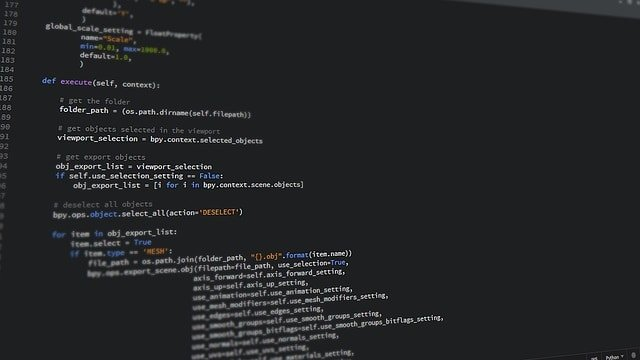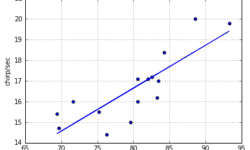## How Is Python Different Than Any Other Programming Languages?Among many of the programming languages available today, Python is one such option that is in high demand by now. The increasing demand for the language is because it is entirely different than many other programming languages available in the market. One such difference or rather benefit is that Python is quite easy to understand and relatively simple to learn. Do you want to learn Python from scratch? Then Intellipaat Python Course is for you. Typically, selecting a proper programming language depends on many factors, such as training, availability, cost, emotional attachment, and prior investments. But these factors are also variable, and hence there are other factors on which the selection of the correct programming language highly depends. Some of the programming languages available in the market now are Java, PHP, C++, Perl, Ruby, Javascript, TCL, Smalltalk, and many others. Python is very much different than these languages in many ways.

## Linear regression – learning algorithm with PythonIn this post, we will demystify the learning algorithm of linear regression. We will analyze the simplest univariate case with single feature X wherein the previous example was temperature and output was cricket chirps/sec. Let’s use the same data with crickets to build learning algorithm and see if it produces a similar hypothesis as in excel. As you may already know from this example, we need to find linear equation parameters θ0 and θ1, to fit line most optimally on the given data set: y = θ0 + θ1 x x here is a feature (temperature), and y – output value (chirps/sec). So how are we going to find parameters θ0 and θ1? The whole point of the learning algorithm is doing this iteratively. We need to find optimal θ0, and θ1 parameter values, so that approximation line error from the plotted training set is minimal. By doing successive corrections to randomly selected parameters we can find an optimal solution. From statistics, you probably know the Least Mean Square (LMS) algorithm. It uses gradient-based method of steepest descent.